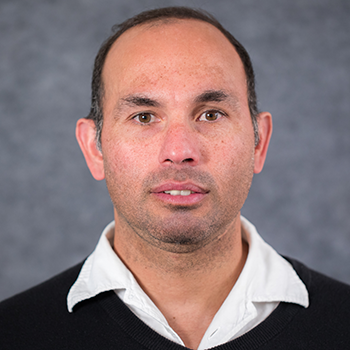# Gilberto Gonzalez-Parra, PhD

Associate Professor

Mathematics

### Research Areas

My main research interests are in the area of mathematical modeling. In particular, I have been involved in the mathematical modeling of processes that emerge from life sciences such as epidemics, diseases, social behaviors, viruses and cellular systems. It is important to remark that, life sciences are expanding and the related topics are increasing exponentially. The life sciences problems nowadays have become a huge source of mathematical problems for different areas of mathematics. Thus, it has been necessary to create interdisciplinary groups to solve the challenge problems that arise.

The main mathematical tools that I have been using for research are nonlinear dynamical systems, differential equations, numerical methods, discrete mathematics, probability, stochastic processes, scientific computing, and numerical analysis. In particular I have applied these tools to study the dynamics of influenza, Chagas, RSV, Toxoplasmosis, obesity, finance and others.